# Python For GCSE ASCII Art Sprites

## Introduction

This project is all about looking for compact ways to store the information needed to produce ASCII art images.

## Step 1 - Getting The Binary Representation Of A Number

All data in a computer is ultimately stored as a binary pattern. More often than not, the layers of abstraction in user interfaces and programming languages obscure this fact from us. If we can find an efficient way to get the binary representation of a denary number, we can put characters where the 1s are, spaces for the 0s and start to buid up an image of sorts.

The technique I prefer for this uses the bitwise operator and. Bitwise operators perform logic operations between integers. The AND logic gate returns a 1 if both of the inputs are 1.

To appreciate how this works, consider the following,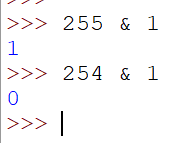Let's write out these operations in pure binary and see if that helps us make sense of it.

```255 - 11111111 001 - 00000001 AND - 00000001 254 - 11111110 001 - 00000001 AND - 00000000```

The AND operation works by comparing the digits in each column. If they are both 1s, then the result will have a 1 in that column. If we do the AND with 1, then we make a pattern with 0s in every column except the units. The result we get is 1 or 0, depending on whether or not there is a 1 in that column. This means that we have a way of telling if a number has a 1 as the rightmost bit.

The other operation we need is a logical shift. Logical shifts can shift all of the digits of an integer a specific number of place values to the left or right. If we do this repeatedly, we can determine the value of all of the bits in our number.

Working from right to left, that means,

```def tobinary(n):
bits = []
for i in range(8):
bits.append(n & 1)
n = n >> 1
return bits
```

Testing this out, we get the following,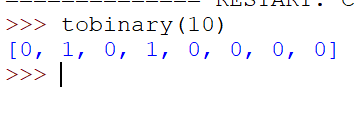This is right. The least significant bit (LSB) comes first and we are working from the right end of our number to the left.

We can refine the algorithm a little and remove a line.

```def tobin(n):
bits = []
for i in range(8):
bits.append((n>>i) & 1)
return bits
```

Now we have this, we can reverse it to make it work from left to right, most significant bit (MSB) first.

```def tobin(n):
bits = []
for i in range(7,-1,-1):
bits.append((n>>i) & 1)
return bits
```

We can reduce the code considerably if we sneak in a list comprehension,

```def tobin(n):
return [(n>>i) & 1 for i in range(7,-1,-1)]
```

## Step 2 - Defining Patterns

Our next step is to get some data together for a sprite. We're going to work with 8x8 sprites. That makes a convenient square. I'm going to use a spreadsheet to make the process a little easier.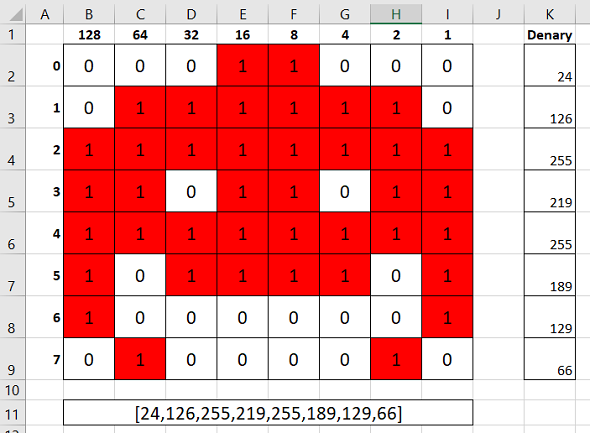This didn't take long. Start by setting up the column sizes, adding borders. Do the row numbers in column A and type the binary place values into Row 1. I used conditional formatting on the grid to make the cells have a red fill when they contain a 1. This makes it easier to see the image you are designing. The formula I used in cell K2 was,

`=B2*\$B\$1+C2*\$C\$1+D2*\$D\$1+E2*\$E\$1+F2*\$F\$1+G2*\$G\$1+H2*\$H\$1+I2*\$I\$1`

I filled this formula down for the other rows of the grid.

I also used a formula in cell B11 to put the numbers into the format I need for a Python list. It was,

`=CONCAT("[",K2,",",K3,",",K4,",",K5,",",K6,",",K7,",",K8,",",K9,"]")`

If you use the same cells as I have, you can copy these formulas into your spreadsheet.

## Step 3 - Drawing The Image

Our next step is to write a subroutine that can draw a character from the list that we created. We will also use a parameter for the character we draw.

Here is one way to do that,

```alien = [24,126,255,219,255,189,129,66]

def tobin(n):
return [(n>>i) & 1 for i in range(7,-1,-1)]

# draw a sprite using lst for the data and pix for the pixels
def draw_sprite(lst,pix):
for r in lst:
row = tobin(r)
for bit in row:
if bit==1:
print(pix, end="")
else:
print(" ", end="")
print()
```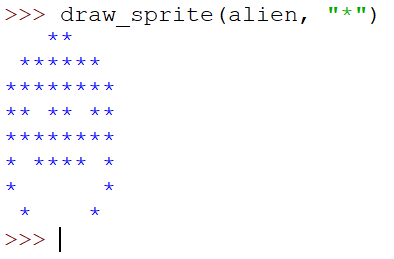Another sneaky use of a list comprehension and we can make this a little more compact.

```def draw_sprite2(lst, pix):
result = ""
for r in lst:
row = [pix if (r>>i) & 1 else " " for i in range(7,-1,-1)]
result += "".join(row) + "\n"
print(result)
```

Testing this gives us,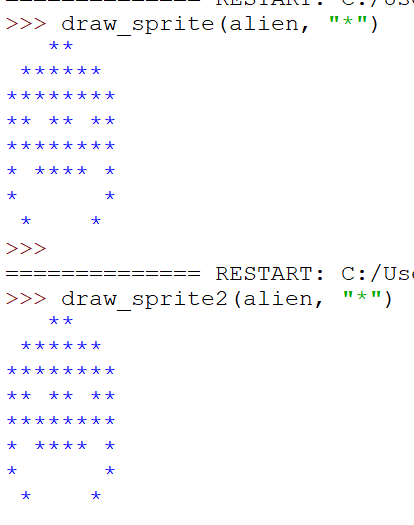This puts a line break at the end. If you didn't want to have that, make result into a list of strings. Join the strings with the line break at the end.

## Next Steps

With the above completed, there are a few possibilities that we can explore.

You could work out the data for a font. You could then read in a string and output it in your ASCII art font. To do that, make a list of lists. Start with upper case only to test and do the alphabet in order. When you read a character from the string you want to print, work out its ASCII number. A capital 'A' has the ASCII number 65. Subtract 65 from a capital number and it will give you a 0, the first item in your list.

Another thing you might do is define the ASCII art for something you want to output for a different program. For example, you could do the images you want to use for a hangman game. The format we are using here is nice and compact.

A trickier adaptation to make, but one that is worth the effort, is to work out how to process the rows of several sprites at the same time. That is what you would need to do if you wanted ASCII art letters side by side.